We think you are located in United States. Is this correct?

# 21.2 Reference frame

## 21.2 Reference frame (ESAGT)

The first thing to focus on when studying motion of an object or person is their position. The word position describes your location (where you are). However, saying that you are here or there is meaningless, you have to use known points (reference points) to help specify your position.

For example, if you were in a classroom and wanted to tell a classmate where you were standing you would first give them a reference point. The reference point might be the classroom door. You would then be able to say that you are $$\text{2}$$ $$\text{m}$$ from the doorway. This still doesn't give your position precisely. We need to provide a reference point and a coordinate system to use to define the location precisely.Then you can say that you are, for example, $$\text{2}$$ $$\text{m}$$ from the door directly inside the classroom. The classroom door is a reference point and inside/outside is the coordinate system you have chosen. A frame of reference or reference frame is reference point which serves as the origin for a coordinate system. The coordinate system can be up or down, inside or outside, left or right or even forward or backward. These are all examples that define a $$\text{1}$$-dimensional coordinate system. We choose one of the directions as the positive direction.

Frame of reference

A frame of reference is a reference point combined with a set of directions.

A graphical representation of a $$\text{1}$$-dimensional frame of reference:

You can define different frames of reference for the same problem but the outcome, the physical results, will be the same. For example, a boy is standing still inside a train as it pulls out of a station. Both you and the boy define your location as the point of reference and the direction train is moving as a where you are standing as the point of reference and the direction the train is moving in as forward.

You are standing on the platform watching the train move from left to right. To you it looks as if the boy is moving from left to right, because relative to where you are standing (the platform), he is moving. According to the boy, and his frame of reference (the train), he is not moving.

A frame of reference must have an origin (where you are standing on the platform) and at least a positive direction. The train was moving from left to right, making to your right positive and to your left negative. If someone else was looking at the same boy, his frame of reference will be different. For example, if he was standing on the other side of the platform, the boy will be moving from right to left.For this chapter, we will only use frames of reference in the $$x$$-direction. By doing this we restrict ourselves to one dimensional motion. We can use the sign of the position value (positive or negative) to indicate the direction relative to the origin.

One dimensional motion

An object is constrained to move back and forth along a line.

For example the blue dot in the figure below can only move along the $$x$$-axis.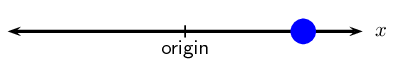### Position (ESAGU)

Position

Position is a measurement of a location, with reference to an origin.

Quantity: Position ($$x$$) Unit name: metre Unit symbol: $$\text{m}$$

A position is a measurement of a location within a reference frame. This means that positions can be negative or positive depending on the choice for the reference frame's coordinate system.

Depending on which reference point we choose, we can say that the school is $$\text{300}$$ $$\text{m}$$ from Kosma's house (with Kosma's house as the reference point or origin) or $$\text{500}$$ $$\text{m}$$ from Kevin's house (with Kevin's house as the reference point or origin).The shop is also $$\text{300}$$ $$\text{m}$$ from Kosma's house, but in the opposite direction as the school. When we choose a reference point, we have a positive direction and a negative direction. If we choose the direction towards the school as negative, then the direction towards the shop is positive. A negative direction is always opposite to the direction chosen as positive.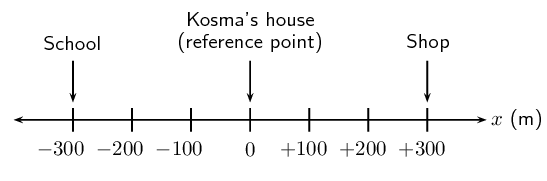The origin is at Kosma's house and the position of the school is $$-\text{300}$$ $$\text{m}$$. Positions towards the left are defined as negative and positions towards the right are defined as positive.

Note that we could also choose the positive direction to be towards the school. In this case Kosma's house is still $$\text{300}$$ $$\text{m}$$ away from the school, but it is now in the positive direction.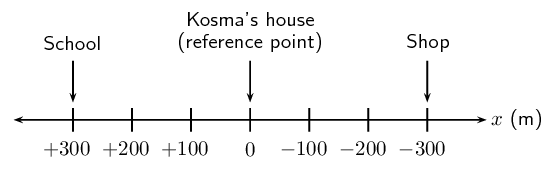The origin is at Kosma's house and the position of the school is $$\text{+300}$$ $$\text{m}$$. Positions towards the left are defined as positive and positions towards the right are defined as negative.

## Reference points

Divide into groups of $$\text{5}$$ for this activity. On a straight line, choose a reference point. Since position can have both positive and negative values, discuss the advantages and disadvantages of choosing

1. either end of the line,

2. the middle of the line. (This reference point can also be called “the origin”.)

Stand in a straight line, take turns choosing a different group member as the origin. Let the group member choose which direction along the line is positive. Everyone should then try to define their position. You don't need to measure your position very precisely but can just approximate it. It is important to understand whether your position is positive or negative for each difference origin and choice of coordinate system.

Notice that your position is different every time but that you didn't actually move. How you write an answer might be affected by the choice of a coordinate system but physical processes should never be affected.

## Position

Textbook Exercise 21.1

Write down the positions for objects at $$A$$, $$B$$, $$D$$ and $$E$$. Do not forget the units.

Solution not yet available

Write down the positions for objects at $$F$$, $$G$$, $$H$$ and $$J$$. Do not forget the units.

Solution not yet available

There are $$\text{5}$$ houses on Newton Street, $$A$$, $$B$$, $$C$$, $$D$$ and $$E$$. For all cases, assume that positions to the right are positive.

1. Draw a frame of reference with house $$A$$ as the origin and write down the positions of houses $$B$$, $$C$$, $$D$$ and $$E$$.

2. You live in house $$C$$. What is your position relative to house $$E$$?

3. What are the positions of houses $$A$$, $$B$$ and $$D$$, if house $$B$$ is taken as the reference point?

Solution not yet available

### Displacement and distance (ESAGV)

Distance

Distance is the total length of the path taken in going from the initial position, $$\vec{x}_{i}$$, to the final position, $$\vec{x}_{f}$$. Distance is a scalar.

Quantity: Distance ($$D$$) Unit name: metre Unit symbol: $$\text{m}$$

The symbol Δ is read out as delta. Δ is a letter of the Greek alphabet and is used in Mathematics and Science to indicate a change in a certain quantity, or a final value minus an initial value. For example, $$\Delta x$$ means change in $$x$$ while $$\Delta t$$ means change in $$t$$.

In the simple map below you can see the path that winds because of a number of hills from a school to a nearby shop. The path is shown by a dashed line. The initial point, $$\vec{x}_{i}$$, is the school and the final point, $$\vec{x}_{f}$$, is the shop.

Distance is the length of dashed line. It is how far you have to walk along the path from the school to the shop.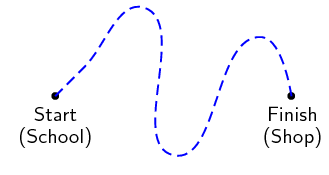Displacement

Displacement is the change in an object's position. It is a vector that points from the initial position ($$\vec{x}_{i}$$) to the final position ($$\vec{x}_{f}$$).

Quantity: Displacement ($$\Delta \vec{x}$$) Unit name: metre Unit symbol: $$\text{m}$$

The displacement of an object is defined as its change in position (final position minus initial position). Displacement has a magnitude and direction and is therefore a vector. For example, if the initial position of a car is $$\vec{x}_{i}$$ and it moves to a final position of $$\vec{x}_{f}$$, then the displacement is:

$\Delta \vec{x} = \vec{x}_{f} - \vec{x}_{i}$

To help visualise what the displacement vector looks like think back to the tail-to-head method. The displacement is the vector you add to the initial position vector to get a vector to the final position.

However, subtracting an initial quantity from a final quantity happens often in Physics, so we use the shortcut Δ to mean final - initial. Therefore, displacement can be written:

$\Delta \vec{x} = \vec{x}_{f} - \vec{x}_{i}$

The following diagram illustrates the concept of displacement:

For example, if you roll a ball $$\text{5}$$ $$\text{m}$$ along a floor, in a straight line, then its displacement is $$\text{5}$$ $$\text{m}$$, taking the direction of motion as positive, and the initial position as $$\text{0}$$ $$\text{m}$$.

The words initial and final will be used very often in Physics. Initial refers to the situation in the beginning of the description/problem and final to the situation at the end. It will often happen that the final value is smaller than the initial value, such that the difference is negative. This is ok!

Displacement does not depend on the path travelled, but only on the initial and final positions. We use the word distance to describe how far an object travels along a particular path.

If we go back to the simple map repeated below you can see the path as before shown by a dashed line.

We will use $$D$$ in this book, but you may see $$d$$ used in other books.

We use the expression 'as the crow flies' to mean a straight line between two points because birds can fly directly over many obstacles.

Distance is the length of dashed line. The displacement is different. Displacement is the straight-line distance from the starting point to the endpoint – from the school to the shop in the figure as shown by the solid arrow.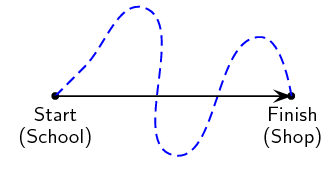#### Illustration of distance and displacement

If we use the same situation as earlier we can explore the concepts in more detail. Consider our description of the location of the houses, school and the shop.Komal walks to meet Kevin at his house before walking to school. What is Komal's displacement and what distance did he cover if he walks to school via Kevin's house?

Komal covers a distance of $$\text{400}$$ $$\text{m}$$ to Kevin's house and another $$\text{500}$$ $$\text{m}$$ from Kevin's house to the school. He covers a total distance of $$\text{900}$$ $$\text{m}$$. His displacement, however, is only $$\text{100}$$ $$\text{m}$$ towards the school. This is because displacement only looks at the starting position (his house) and the end position (the school). It does not depend on the path he travelled.

To calculate his distance and displacement, we need to choose a reference point and a direction. Let's choose Komal's house as the reference point, and towards Kevin's house as the positive direction (which means that towards the school is negative). We would do the calculations as follows:

\begin{align*} \text{Distance } (D) & = \text{path travelled } \\ & = \text{400}\text{ m} + \text{500}\text{ m} \\ & = \text{900}\text{ m} \end{align*}\begin{align*} \text{Displacement } \left(\Delta \vec{x}\right) & = \vec{x}_{f} - \vec{x}_{i} \\ & = -\text{100}\text{ m} + \text{0}\text{ m} \\ & = -\text{100}\text{ m} \\ & = \text{100}\text{ m} \text{ (in the negative } x \text{ direction)} \end{align*}

Very often in calculations you will get a negative answer. For example, Komal's displacement in the example above, is calculated as $$-\text{100}$$ $$\text{m}$$. The minus sign in front of the answer means that his displacement is $$\text{100}$$ $$\text{m}$$ in the opposite direction (opposite to the direction chosen as positive in the beginning of the question). When we start a calculation we choose a frame of reference and a positive direction. In the first example above, the reference point is Komal's house and the positive direction is towards Kevin's house. Therefore Komal's displacement is $$\text{100}$$ $$\text{m}$$ towards the school. Notice that distance has no direction, but displacement has a direction.

Kevin walks to school with Komal and after school walks back home. What is Kevin's displacement and what distance did he cover? For this calculation we use Kevin's house as the reference point. Let's take towards the school as the positive direction.

\begin{align*} \text{Distance } (D) & = \text{ path travelled} \\ & = \text{500}\text{ m} + \text{500}\text{ m} \\ & = \text{1 000}\text{ m} \end{align*}\begin{align*} \text{Displacement } \left(\Delta \vec{x}\right) & = \vec{x}_{f} - \vec{x}_{i} \\ & = \text{0}\text{ m} + \text{0}\text{ m} \\ & = \text{0}\text{ m} \end{align*}

It is possible to have a displacement of $$\text{0}$$ $$\text{m}$$ and a distance that is not $$\text{0}$$ $$\text{m}$$. This happens whenever you end at the same point you started.

#### Differences between distance and displacement

The differences between distance and displacement can be summarised as:

 Distance Displacement 1. depends on the path 1. independent of path taken 2. always positive 2. can be positive or negative 3. is a scalar 3. is a vector

## Frames of reference, displacement and distance

Textbook Exercise 21.2

Use this figure to answer the following questions.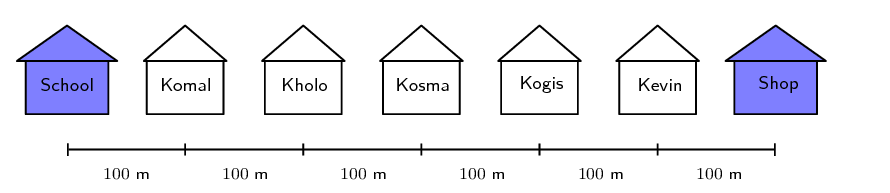1. Kogis walks to Kosma's house and then to school, what is her distance and displacement?

2. Kholo walks to Kosma's house and then to school, what is her distance and displacement?

3. Komal walks to the shop and then to school, what is his distance and displacement?

4. What reference point did you use for each of the above questions?

Solution not yet available

You stand at the front door of your house (displacement, $$\Delta \vec{x} = \text{0}\text{ m}$$). The street is $$\text{10}$$ $$\text{m}$$ away from the front door. You walk to the street and back again.

1. What is the distance you have walked?

2. What is your final displacement?

3. Is displacement a vector or a scalar? Give a reason for your answer.

Solution not yet available